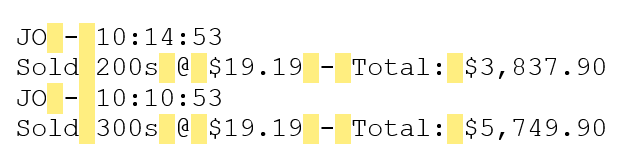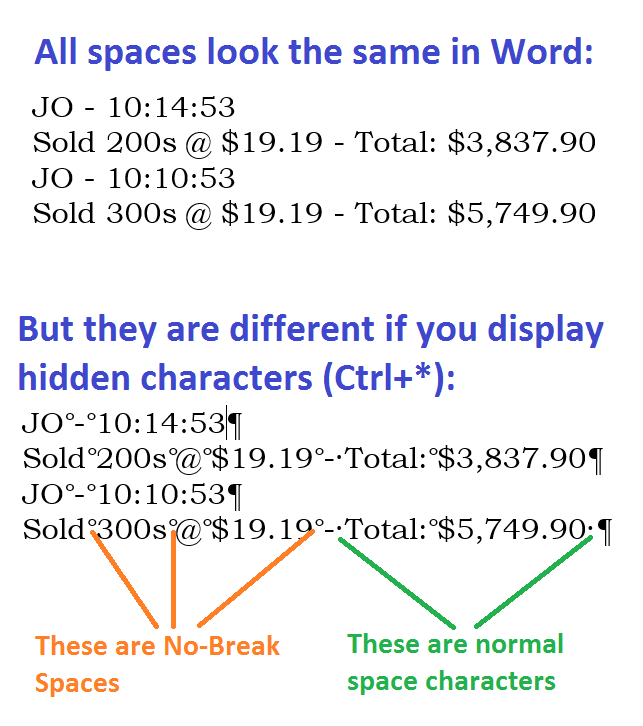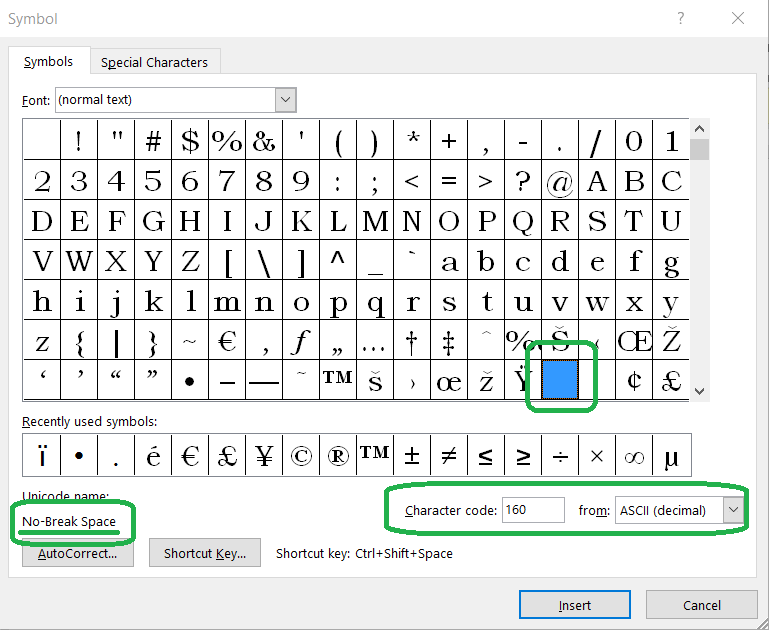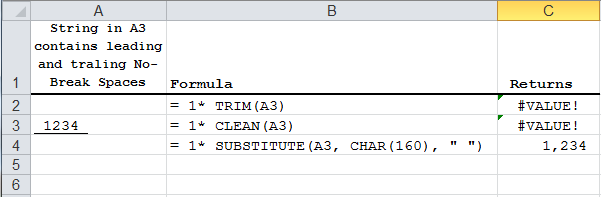# Can’t convert Number into a string in Excel (not all space characters are the same)

Problem started as a simple CAN’T CONVERT NUMBER INTO A STRING in Excel.

But solving this problem revealed a deep difference between character Space and character No-Break Space.

I was copying some text from Internet and pasting it into Excel to extract QTY, Amount and Total.  The ultimate goal is to calculate the commissions, but that is not important here.  Below is an example of text that I would normally copy into Excel:

```JO - 10:14:53
Sold 200s @ \$19.19 - Total: \$3,837.90
JO - 10:10:53
Sold 300s @ \$19.19 - Total: \$5,749.90```

Problem: I can extract quantity 200 into a separate cell, but I can’t do any arithmetic operation on a resulting number.  All the usual tricks as for example multiplying by 1, or using TRIM(), or even using function VALUE() will return the same result – #VALUE!.   Somehow, Excel does not want to recognize resulting 200 as a number.

Before we begin, let’s list lessons learned while resolving this issue:

(01) Space (ASCII 32) and No-Break Space (ASCII 160) are two completely different characters.
(02) Word and Excel behaving differently in handling these two characters.
(03) Excel TRIM() function will not remove No-Break Space from the string.
(04) Word will search and find both Space and No-Break Space as the same character.
(05) To handle No-Break Space in Excel use function SUBSTITUTE()
(06) You can determine, what special character is currently used in the string by using Word insert special symbol dialog box.

## First, I needed to fix the problem, why Excel is not recognizing 200 as a number?

My formula to extract “200” was:

`=TRIM(MID(B48,FIND(" ",B48),FIND("s @",B48)-FIND(" ",B48)))*1`

Original test string was “Sold 200s @ \$19.19 – Total: \$3,837.90″ and it is located in cell B48.

Function FIND(” “,B48) returns 5.

Function FIND(“s @”,B48) returns 9.

I noticed that I have to cut and paste empty space from the original string into FIND() function parameter for it to work.  If I just type blank character between quotation marks (like this ” “) function will return not 5, but 21.  For some reason, function FIND() thinks that first blank character is a character before word ” Total”.

Returning to original long formula:

`=TRIM(MID(B48,FIND(" ",B48),FIND("s @",B48)-FIND(" ",B48)))*1`

MID() function is extracting a sub-string starting from position 5 and uses the length of 4 (9-5).  I noticed that I am actually extracting not “200”, but ” 200″ with a special blank character in front.  To solve my immediate Excel problem, I decided to start with position 6 (5+1) and extract only 3 characters (9-1-5).  I modified the formula to look like this:

`=TRIM(MID(B48,FIND(" ",B48)+1,FIND("s @",B48)-1-FIND(" ",B48)))*1`

That did it for Excel.  Now I could work with substring”200″ as a number and apply arithmetic operations to it.

## Second, what is hiding behind special space characters?

But the bigger problem remained: what is going on with the string that I copy and paste from Internet?  What special character preventing TRIM() and FIND() functions to work properly?

I pasted the string in Word, and used search for a Space character, and Word found them as expected without discriminating:But if I try to type a normal space here after word “Sold” and paste back into Excel, once again the formula will no longer work and return #VALUE!, because it is set to FIND that special space from web page, not the normal space from keyboard.

Next I decided to use Display Hidden Characters in Word to see what is going on that do not detect on the screen:OK. So now I can clearly see, that I am dealing with an unusual character in my copy and paste string. Now let me see what this character actually represents.

In Word, I selected the mysterious blank space after word “Sold” and used insert special character dialog (image below). Word automatically recognizes selected special character and highlights corresponding cell in the matrix of special characters:Here it is.  We are dealing with space character that is needed on web pages to keep certain word together and prevent string to break in the middle.  Ok.  That is good.  Next logical question – can I write a formula in Excel that will FIND both regular space and No-Break Space and return string “200” reliably?Functions TRIM() or CLEAN() can’t help you (image above) .  The answer is function SUBSTITUTE() that can be instructed to replace No-Break Spaces (ASCII 160) with regular spaces:

`=IF(B48="", "", SUBSTITUTE(B48, CHAR(160), " "))`

Now I am storing a new string without No-Break Spaces at cell E48.

Now I can simplify the formula to extract string “200”:

`=IF(E48="","",1*MID(E48,FIND(" ",E48),FIND("s @",E48)-FIND(" ",E48)))`